# PSEB 12th Class Physics Solutions Chapter 7 Alternating Current

Punjab State Board PSEB 12th Class Physics Book Solutions Chapter 7 Alternating Current Textbook Exercise Questions and Answers.

## PSEB Solutions for Class 12 Physics Chapter 7 Alternating Current

PSEB 12th Class Physics Guide Alternating Current Textbook Questions and Answers

Question 1.
A 100 Ω resistor is connected to a 220 V, 50 Hz ac supply.
(a) What is the rms value of current in the circuit?
(b) What is the net power consumed over a full cycle?
The given voltage of 220 V is the rms or effective voltage.
Given Vrms = 220 V, v = 50 Hz, R = 100 Ω
(a) RMS value of current,
Irms = $$\frac{V_{r m s}}{R}$$ = $$\frac{220}{100}$$ = 2.2 A
Net power consumed, P = I2rmsR
= (2.20)2 × 100 = 484 W

Question 2.
(a) The peak voltage of an ac supply is 300 V. What is the rms voltage?
(b) The rms value of current in an ac circuit is 10 A. What is the peak current?
(a) Given, V0 = 300 V
Vrms = $$\frac{V_{0}}{\sqrt{2}}=\frac{300}{\sqrt{2}}$$ = 150√2 ≈ 212 V

(b) Given, Irms = 10 A
I0 = Irms √2 = 10 × 1.41 = 14.1 AQuestion 3.
A 44 mH inductor is connected to 220 V, 50 Hz ac supply. Determine the rms value of the current in the circuit.
Inductance of inductor, L = 44 mH = 44 × 10-3 H
Supply voltage, V = 220 V
Frequency, v = 50 Hz
Angular frequency, ω = 2 πv
Inductive reactance, XL = ωL = 2πvL × 2π × 50 × 44 × 10-3Ω
rms value of current is given as
I = $$\frac{V}{X_{L}}$$ = $$\frac{220}{2 \pi \times 50 \times 44 \times 10^{-3}}$$ = 15.92 A
Hence, the rms value of current in the circuit is 15.92 A.

Question 4.
A 60 μF capacitor is connected to a 110 V, 60 Hz ac supply. Determine the rms value of the current in the circuit.
Capacitance of capacitor, C = 60μF = 60 × 10-6F
Supply voltage, V = 110 V
Frequency, v = 60 Hz
Angular frequency, ω = 2 πv
Capacitive reactance,
XC = $$\frac{1}{\omega C}$$ = $$\frac{1}{2 \pi v C}$$ = $$\frac{1}{2 \pi \times 60 \times 60 \times 10^{-6}}$$Ω
rms value of current is given as
I = $$\frac{V}{X_{C}}$$ = $$\frac{110}{\frac{1}{2 \pi \times 60 \times 60 \times 10^{-6}}}$$
= 110 × 2 × 3.14 × 3600 × 10-6
= 2.49 A
Hence, the rms value of current in the circuit is 2.49 A.Question 5.
In Exercises 7.3 and 7.4, what is the net power absorbed by each circuit over a complete cycle. Explain your answer.
In the inductive circuit,
rms value of current, I = 15.92 A
rms value of voltage, V = 220 V
Hence, the net power absorbed by the circuit, can be obtained by the relation,
P = VIcosΦ
where,
Φ = Phase difference between V and I.
For a pure inductive circuit, the phase difference between alternating voltage and current is 90°i. e., Φ = 90°
Hence, P = 0 i. e., the net power is zero.

In the capacitive circuit,
rms value of current, I = 2.49 A
rms value of voltage, V = 110 V
Hence, the net power absorbed by the circuit, can be obtained as
P = VIcosΦ
For a pure capacitive circuit, the phase difference between alternating voltage and current is 90°i. e., Φ = 90 °
Hence, P = 0 i. e., the net power is zero.

Question 6.
Obtain the resonant frequency ωr of a series LCR circuit with L = 2.0 H, C = 32 μF and R = 10 Ω. What is the Q-value of this circuit?
Resonant frequency,
ωr = $$\frac{1}{\sqrt{L C}}$$ = $$\frac{1}{\sqrt{2.0 \times 32 \times 10^{-6}}}$$
= $$\frac{1}{8}$$ × 103 = 125 rads-1
Q = $$\frac{\omega_{r} L}{R}$$ = $$\frac{125 \times 2.0}{10}$$ = 25

Question 7.
A charged 30 μF capacitor is connected to a 27 mH inductor.
What is the angular frequency of free oscillations of the circuit?
Capacitance of the capacitor, C = 30 μF = 30 × 10-6 F,
Inductance of the inductor, L = 27 mH = 27 × 10-3H
Angular frequency is given as
ωr = $$\frac{1}{\sqrt{L C}}$$
= $$\frac{1}{\sqrt{27 \times 10^{-3} \times 30 \times 10^{-6}}}$$
= $$\frac{1}{9 \times 10^{-4}}=\frac{10^{4}}{9}$$
Hence, the angular frequency of free oscillations of the circuit is 1.11 × 103 rad/s.Question 8.
Suppose the initial charge on the capacitor in Exercise 7.7 is 6 mC. What is the total energy stored in the circuit initially? What is the total energy at later time?
Capacitance of the capacitor, C = 30 μF = 30 × 10-6F
Inductance of the inductor, L = 27 mH = 27 × 10-3 H
Charge on the capacitor, Q = 6 mC = 6 × 10-3 C
Total energy stored in the capacitor can be calculated as
E = $$\frac{1}{2} \frac{Q^{2}}{C}$$ = $$\frac{1}{2} \frac{\left(6 \times 10^{-3}\right)^{2}}{\left(30 \times 10^{-6}\right)}$$
= $$\frac{36 \times 10^{-6}}{2\left(30 \times 10^{-6}\right)}$$
= $$\frac{6}{10}$$ = 0.6 J
Total energy at a later time will remain the same because energy is shared between the capacitor and the inductor.

Question 9.
A series LCR circuit with R = 20 Ω, L = 1.5 H and C = 35 μF is connected to a variable frequency 200 V ac supply. When the frequency of the supply equals the natural frequency of the circuit, what is the average power transferred to the circuit in one complete cycle?
When frequency of supply is equal to natural frequency of circuit, then resonance is obtained. At resonance XC = XL
⇒ Impedance, Z = $$\sqrt{R^{2}+\left(X_{C}-X_{L}\right)^{2}}$$
= R = 20Ω
Current in circuit,
Irms = $$\frac{V_{r m s}}{R}$$ = $$\frac{200}{20}$$ = 10A
Power factor
cosΦ = $$\frac{R}{Z}=\frac{R}{R}$$ = 1
∴ Average power pav = Vrms Irms cosΦ = Vrms Irms
= 20 × 10 = 2000 W = 2 kWQuestion 10.
A radio can tune over the frequency range of a portion of MW broadcast band : (800 kHz to 1200 kHz). If its LC circuit has an effective inductance of 200 μH, what must be the range of its variable capacitor?
[Hint: For timing, the natural frequency i. e., the frequency of free oscillations of the LC circuit should be equal to the frequency of the radiowave.]
The range of frequency (v) of the radio is 800 kHz to 1200 kHz
Lower tuning frequency, v1 = 800 kHz = 800 × 103 Hz
Upper tuning frequency, v2 = 1200 kHz = 1200 × 106 Hz
Effective inductance of circuit, L = 200 μH = 200 × 10-6 H
Capacitance of variable capacitor for v1 is given as
C1 = $$\frac{1}{\omega_{1}^{2} L}$$
where, ω1 = Angular frequency for capacitor C1
= 2 πv1
= 2 π × 800 × 103 rad/s
∴ C1 = $$\frac{1}{\left(2 \pi \times 800 \times 10^{3}\right)^{2} \times 200 \times 10^{-6}}$$
= 197.8 × 10-12F
= 197.8 pF
Capacitance of variable capacitor for v2 is given as
C2 = $$\frac{1}{\omega_{2}^{2} L}$$
where,
ω2 = Angular frequency for capacitor C2
= 2πv2
= 2 π × 1200 × 103 rad/s
∴ C 2 = $$\frac{1}{\left(2 \pi \times 1200 \times 10^{3}\right)^{2} \times 200 \times 10^{-6}}$$
= 87.95 × 10-12 F = 87.95 pF
Hence, the range of the variable capacitor is from 87.95 pF to 197.8 pF.

Question 11.
Figure 7.21 shows a series LCR circuit connected to a variable frequency 230 V source. Z, = 5.0H, C = 80 μF, R = 40Ω.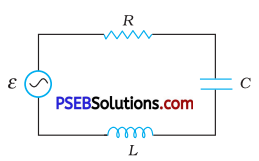(a) Determine the source frequency which drives the circuit in resonance.
(b) Obtain the impedance of the circuit and the amplitude of current at the resonating frequency.
(c) Determine the rms potential drops across the three elements of the circuit. Show that the potential drop across the LC combination is zero at the resonating frequency.
Given, the rms value of voltage Vrms = 230 V
Inductance L = 5H
Capacitance C = 80 μF = 80 × 10-6 F
Resistance R = 40 Ω

(a) For resonance frequency of circuit
ωr = $$\frac{1}{\sqrt{L C}}$$ = $$\frac{1}{\sqrt{5 \times 80 \times 10^{-6}}}$$ = 50 rad/s
Source frequency at resonance, then
v0 = $$\frac{\omega_{0}}{2 \pi}$$ = $$\frac{50}{2 \times 3.14}$$ = 7.76 Hz

(b) At the resonant frequency, XL = XC
So, impedance of the circuit Z = R
∴ Impedance Z = 40 Ω
The rms value of current in the circuit
Irms = $$\frac{V_{r m s}}{Z}$$ = $$\frac{230}{40}$$ = 5.75 A
Amplitude of current, I0 = Irms √2
= 5.75 × √2 = 8.13 A

(c) The rms potential drop across I,
VL = Irms × XL = Irms × ωrL
= 5.75 × 50 × 5 = 1437.5V
The rms potential drop across R
VR = Irms R = 5.75 × 40 = 230 V
The rms potential drop across C,
VC = Irms × XC = Irms × $$\frac{1}{\omega_{r} C}$$
= 5.75 × $$\frac{1}{50 \times 80 \times 10^{-6}}$$
= 1437.5V
Potential drop across LC combinations
= Irms(XL – XC)
= Irms (XL – XL) = 0
(∵ XL = XC in resonance)Question 12.
An LC circuit contains a 20 mH inductor and a 50 μF capacitor with an initial charge of 10 mC. The resistance of the circuit is negligible. Let the instant the circuit is closed be t = 0.
(a) What is the total energy stored initially? Is it conserved during LC oscillations?
(b) What is the natural frequency of the circuit?
(c) At what time is the energy stored (i) completely electrical (Lestored in the capacitor)? (ii) completely magnetic (i.e., stored in the inductor)?
(d) At what times is the total energy shared equally between the inductor and the capacitor?
(e) If a resistor is inserted in the circuit, how much energy is eventually dissipated as heat?
Inductance of the inductor, L = 20 mH = 20 × 10-3H
Capacitance of the capacitor, C = 50 μF = 50 × 10-6 F
Initial charge on the capacitor, Q = 10 mC = 10 × 10-3C

(a) Total energy stored initially in the circuit is given as
E = $$\frac{1}{2} \frac{Q^{2}}{C}$$
= $$\frac{\left(10 \times 10^{-3}\right)^{2}}{2 \times 50 \times 10^{-6}}=\frac{10^{-4}}{10^{-4}}$$ = 1J
Hence, the total energy stored in the LC circuit will be conserved because there is no resistor connected in the circuit.

(b) Natural frequency of the circuit is given by the relation,
v = $$\frac{1}{2 \pi \sqrt{L C}}$$
= $$\frac{1}{2 \pi \sqrt{20 \times 10^{-3} \times 50 \times 10^{-6}}}$$
= $$\frac{10^{3}}{2 \pi}$$ = 159.24 Hz
Natural angular frequency,
ωc = $$\frac{1}{\sqrt{L C}}$$ = $$\frac{1}{\sqrt{20 \times 10^{-3} \times 50 \times 10^{-6}}}$$
= $$\frac{1}{\sqrt{10^{-6}}}$$ = 103 rad/s
Hence, the natural frequency of the circuit is 10 rad/s.

(c) (i) For time period (T = $$\frac{1}{v}$$ = $$\frac{1}{159.24}$$ = 6.28 ms), total charge on the
capacitor at time t,
Q’ = Q cos$$\frac{2 \pi}{T}$$t
For energy stored is electrical, we can write Q’ = Q
Hence, it can be inferred that the energy stored in the capacitor is completely electrical at time, t = 0, $$\frac{T}{2}$$, T, $$\frac{3 T}{2}$$,…

(ii) Magnetic energy is the maximum when electrical energy, Q’ is equal to 0.
Hence, it can be inferred that the energy stored in the capacitor is
completely magnetic at time, t = $$\frac{T}{4}$$, $$\frac{3 T}{4}$$, $$\frac{5 T}{4}$$,….

(d) Q’ = Charge on the capacitor when total energy is equally shared between the capacitor and the inductor at time t.
When total energy is equally shared between the inductor and capacitor,
the energy stored in the capacitor = $$\frac{1}{2}$$ (maximum energy)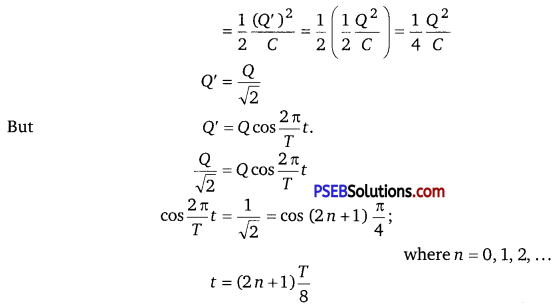Hence, total energy is equally shared between the inductor and the capacitor at time,
t = $$\frac{T}{8}$$, $$\frac{3 T}{8}$$,$$\frac{5 T}{8}$$

(e) If a resistor is inserted in the circuit, then total initial energy is dissipated as heat energy in the circuit. The resistance damps out the LC oscillation.Question 13.
A coil of inductance 0.50 H and resistance 100 Ω is connected to a 240 V, 50 Hz ac supply.
(a) What is the maximum current in the coil?
(b) What is the time lag between the voltage maximum and the current maximum?
Given, L = 0.50 H ,R = 100 Ω, V = 240 V, v = 50 Hz
(a) Maximum (or peak) voltage V0 = V – √2
Maximum current, I0 = $$\frac{V_{0}}{Z}$$
Inductive reactance, XL = ωL = 2πvL
= 2 × 3.14 × 50 × 0.50
= 157 Ω.
Z = $$\sqrt{R^{2}+X_{L}^{2}}$$
= $$\sqrt{(100)^{2}+(157)^{2}}$$ = 186 Ω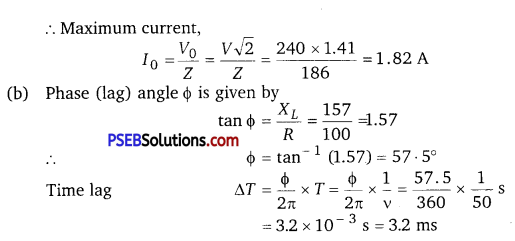Question 14.
Obtain the answers (a) to (b) in Exercise 7.13 if the circuit is connected to a high frequency supply (240 V, 10 kHz). Hence, explain the statement that at very high frequency, an inductor in a circuit nearly amounts to an open circuit. How does an inductor behave in a dc circuit after the steady state?
Inductance of the inductor, L = 0.5 Hz
Resistance of the resistor, R = 100 Ω
Potential of the supply voltage, V = 240 V
Frequency of the supply, v = 10 kHz = 104 Hz
Angular frequency, ω = 2πv = 2 π × 104 rad/s

(a) Peak voltage, V0 = √2 × V = 240√2 V
Maximum current, I0 = $$\frac{V_{0}}{\sqrt{R^{2}+\omega^{2} L^{2}}}$$
= $$\frac{240 \sqrt{2}}{\sqrt{(100)^{2}+\left(2 \pi \times 10^{4}\right)^{2} \times(0.50)^{2}}}$$
= 1.1 × 10-2 A

(b) For phase difference, Φ, we have the relation
tanΦ = $$\frac{\omega L}{R}$$ = $$\frac{2 \pi \times 10^{4} \times 0.5}{100}$$ = 100π
Φ = 89.82° = $$\frac{89.82 \pi}{180}$$ rad
ωt = $$\frac{89.82 \pi}{180}$$
t = $$\frac{89.82 \pi}{180 \times 2 \pi \times 10^{4}}$$ = 25 μs

It can be observed that I0 is very small in this case. Hence, at high frequencies, the inductor amounts to an open circuit.
In a dc circuit, after a steady state is achieved, ω = 0. Hence, inductor L behaves like a pure conducting object.Question 15.
A 100 μF capacitor in series with a 40 Ω resistance is connected to a 110 V, 60 Hz supply.
(a) What is the maximum current in the circuit?
(b) What is the time lag between the current maximum and the voltage maximum?
Capacitance of the capacitor, C = 100 μF = 100 × 10-6 F = 10-4 F
Resistance of the resistor, R = 40 Ω
Supply voltage, V = 110 V
Frequency of oscillations, v = 60 Hz
Angular frequency, co = 2πv = 2π × 60 rad/s = 120 π rad/s
For a RC circuit, we have the relation for impedance as
Z = $$\sqrt{R^{2}+\frac{1}{\omega^{2} C^{2}}}$$
peak voltage V0 = V√2 = 110√2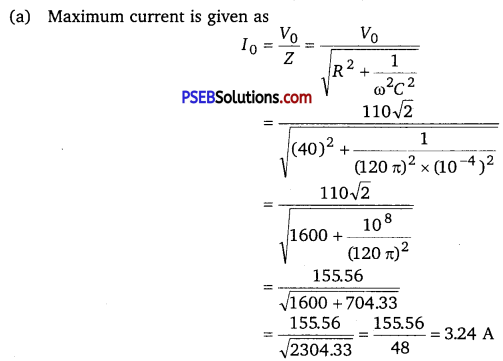(b) In an RC circuit, the voltage lags behind the current by a phase angle of Φ. This angle is given by the relation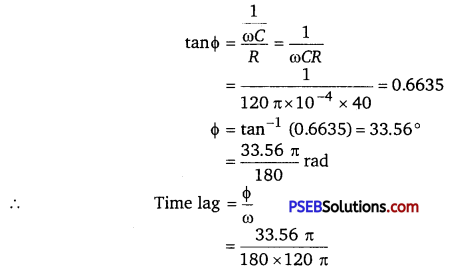= 1.55 × 10-3 s
= 1.55 ms
Hence, the time lag between maximum current and maximum voltage is 1.55 ms.

Question 16.
Obtain the answers to (a) and (b) in Exercise 7.15 if the circuit is connected to a 110 V, 12 kHz supply? Hence, explain the statement that a capacitor is a conductor at very high frequencies. Compare this behaviour with that of a capacitor in a dc circuit after the steady state.
Capacitance of the capacitor, C = 100 μF = 100 × 10-6 F
Resistance of the resistor, R = 40 Ω
Supply voltage, V = 110 V
Frequency of the supply, v = 12 kHz = 12 × 103 Hz
Angular frequency, ω = 2πv = 2 × π × 12 × 103
= 24 π × 103 rad/s
Peak voltage, V0 = V√2 = 110 √2V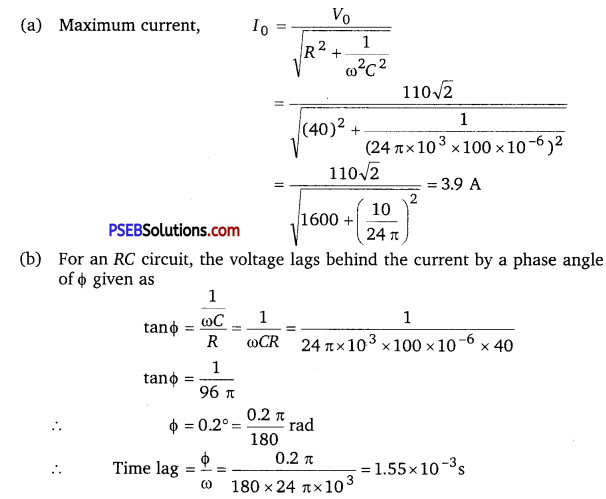= 0.04 μs
Hence, Φ tends to become zero at high frequencies. At a high frequency, capacitor C acts as a conductor.
In a dc circuit, after the steady state is achieved, ω = 0. Hence, capacitor C acts an open circuit.Question 17.
Keeping the source frequency equal to the resonating frequency of the series LCR circuit, if the three elements, L, C and R are arranged in parallel, show that the total current in the parallel LCR circuit is minimum at this frequency. Obtain the current rms value in each branch of the circuit for the elements and source specified in Exercise 7.11 for this frequency.
Here, L = 5.0 H
C = 80 μF = 80 × 10-6 F
R = 40Ω
The effective impedance of the parallel LCR is given by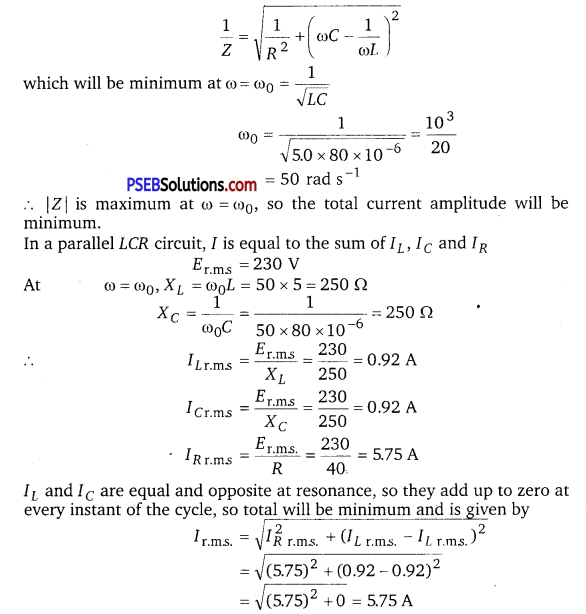Question 18.
A circuit containing a 80 mH inductor and a 60 µF capacitor in series is connected to a 230 V, 50 Hz supply. The resistance of the circuit is negligible.
(a) Obtain the current amplitude and rms values.
(b) Obtain the rms values of potential drops across each element.
(c) What is the average power transferred to the inductor?
(d) What is the average power transferred to the capacitor?
(e) What is the total average power absorbed by the circuit?
[‘Average’ implies ‘averaged over one cycle’.]
Given,
V = 230 V, v = 50 Hz, L = 80 mH = 80 × 10-3 H,
C = 60µF = 60 × 10-6 F

(a) Inductive reactance XL = ωL = 2πvL
= 2 × 3.14 × 50 × 80 × 10-3
= 25.1 Ω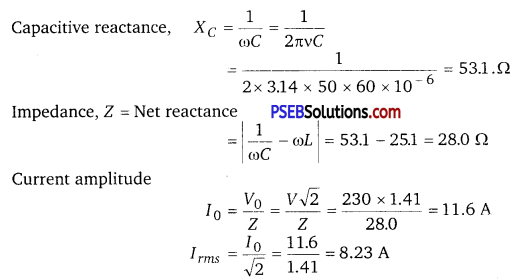(b) RMS value of potential drops across L and C are
VL = XL Irms = 25.1 × 8.23 = 207 V
VC = XC Irms = 53.1 × 8.23 = 437 V
Net voltage = VC – VL = 230 V

(c) The voltage across L leads the current by angle $$\frac{\pi}{2}$$ , therefore, average
power
Pav Vrms Irms cos $$\frac{\pi}{2}$$ = 0 (zero)

(d) The voltage across C lags behind the current by angle $$\frac{\pi}{2}$$,
∴ pav = Vrms Irms cos $$\frac{\pi}{2}$$ = 0

(e) As circuit contains pure I and pure C, average power consumed by LC circuit is zero.Question 19.
Suppose the circuit in Exercise 7.18 has a resistance of 15 Ω. Obtain the average power transferred to each element of the circuit, and the total power absorbed.
Here, R – 15Ω, L = 80 mH = 80 × 10-3 H
C = 60 μF = 60 × 10-6 F.
Er.m.s. = 230 V
v = 50 Hz
> ω = 2πv = 2π × 50 =100 π
Z = impedance of LCR circuit
= $$\sqrt{R^{2}+\left(\omega L-\frac{1}{\omega C}\right)^{2}}$$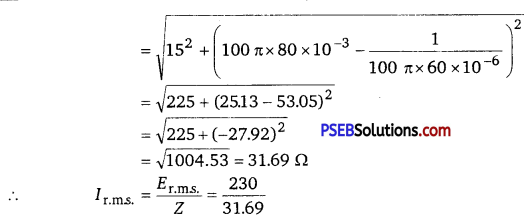= 7.258 = 7.26 A
∴ Average power consumed by R or transferred to R is given by
(Pav)R = I2r.m.s..R = (7.26)2 × 15 = 790.614 W
= 791 W.
Also (Pav)L and (Pav)C be the average power transferred to I and C respectively.
(Pav)L = Er.m.s. . Ir.m.s. cosΦ
Here e.m.f. leads current by $$\frac{\pi}{2}$$
∴ (Pav)L= Er.m.s. . Ir.m.s. cos $$\frac{\pi}{2}$$
= 0
and (Pav )C = = Er.m.s. . Ir.m.s. cosΦ
= 0
( ∵ Φ = $$\frac{\pi}{2}$$ and cos $$\frac{\pi}{2}$$ = 0

If Pav be the total power absorbed in the circuit, then
Pav = (Pav)L + (Pav )C + (Pav )R
= 0 + 0 + 791
= 791 WQuestion 20.
A series LCR circuit with L = 0.12 H, C = 480 nF, R = 23 Ω is connected to a 230 V variable frequency supply.
(a) What is the source frequency for which current amplitude is maximum? Obtain this maximum value.
(b) What is the source frequency for which average power absorbed by the circuit is maximum? Obtain the value of this maximum power.
(c) For which frequencies of the source is the power transferred to the circuit half the power at resonant frequency? What is the current amplitude at these frequencies?
(d) What is the Q-factor of the given circuit?
Inductance, L = 0.12 H
Capacitance, C = 480 nF = 480 × 10-9 F
Resistance, R = 23 Ω
Supply voltage, V = 230 V
Peak voltage is given as V0 = √2V
V0 = √2 × 230 = 325.22 V

(a) Current flowing in the circuit is given by the relation,
I0 = $$\frac{V_{0}}{\sqrt{R^{2}+\left(\omega L-\frac{1}{\omega C}\right)^{2}}}$$
where, I0 = maximum at resonance
At resonance, we have
ωRL – $$\frac{1}{\omega_{R} C}[latex] = 0 where, ωR = Resonance angular frequency ∴ ωR = [latex]\frac{1}{\sqrt{L C}}$$
= $$\frac{1}{\sqrt{0.12 \times 480 \times 10^{-9}}}$$
= $$\frac{10^{5}}{\sqrt{12 \times 48}}=\frac{10^{5}}{24}$$
∴ Resonant frequency; vR = $$\frac{\omega_{R}}{2 \pi}$$ = $$\frac{4166.67}{2 \times 3.14}$$ = 663.48 HZ
and, maximum current (I0)max = $$\frac{V_{0}}{R}$$ = $$\frac{325.22}{23}$$ 14.14 A

(b) Average power absorbed by the circuit is given as
Pav = $$\frac{1}{2}$$I02R

The average power is maximum at ω = ω0 at which I0 = (I0)max
∴ (pav )max = $$\frac{1}{2}$$(I0)2maxR
= $$\frac{1}{2}$$ × (14.14)2 × 23 = 2299.3 W
= 2300 W

(c) The power transferred to the circuit is half the power at resonant frequency.
Frequencies at which power transferred is half, ω = ωR ± Δ ω
= 2π (vR ± Δv)
where, Δω = $$\frac{R}{2 L}$$
= $$\frac{23}{2 \times 0.12}$$ = 95.83 rad/s
Hence, change in frequency, Δ v = $$\frac{1}{2 \pi}$$ Δω = $$\frac{95.83}{2 \pi}$$ = 15.26 Hz
Thus power absorbed is half the peak power at
vR + Δv = 663.48 + 15.26 = 678.74 Hz
and, vR ΔV = 663.48 – 15.26 = 648.22 Hz
Hence, at 648.22 Hz and 678.74 Hz frequencies, the power transferred is half.
At these frequencies, current amplitude can be given as
I’ = $$\frac{1}{\sqrt{2}}$$ × (I0)max = $$\frac{14.14}{\sqrt{2}}=\frac{14.14}{1.414}$$ = 10 A

(d) Q-factor of the given circuit can be obtained using the relation,
Q = $$\frac{\omega_{R} L}{R}$$ = $$\frac{4166.67 \times 0.12}{23}$$ = 21.74
Hence, the Q-factor of the given circuit is 21.74.

Question 21.
Obtain the resonant frequency and Q-factor of a series LCR circuit with L = 3.0 H, C = 27 μF and R = 7.4 Ω. It is desired to improve the sharpness of the resonance of the circuit by reducing its ‘full width at half maximum’ by a factor of 2. Suggest a suitable way.
Inductance, L = 3.0 H
Capacitance, C = 27 μF = 27 × 10-6F
Resistance, R = 7.4 Ω
At resonance, resonant frequency of the source for the given LCR series circuit is given as
ωr = $$\frac{1}{\sqrt{L C}}$$ = $$\frac{1}{\sqrt{3 \times 27 \times 10^{-6}}}$$
$$\frac{10^{3}}{9}$$ = 111.11 rad s-1
Q-factor of the series
Q = $$\frac{\omega_{r} L}{R}$$ = $$\frac{111.11 \times 3}{7.4}$$ = 45.0446
To improve the sharpness of the resonance by reducing its ‘full width at half maximum’ by a factor of 2 without changing cor, we need to reduce R to half i. e., Resistance = $$\frac{R}{2}=\frac{7.4}{2}$$ = 3.7 Ω.Question 22.
(a) In any ac circuit, is the applied instantaneous voltage equal to the algebraic sum of the instantaneous voltages across the series elements of the circuit? Is the same true for rms voltage?

(b) A capacitor is used in the primary circuit of an induction coil.

(c) An applied voltage signal consists of a superposition of a dc voltage and an ac voltage of high frequency. The circuit consists of an inductor and a capacitor in series. Show that the dc signal will appear across C and the ac signal across L.

(d) A choke coil in series with a lamp is connected to a dc line. The lamp is seen to shine brightly. Insertion of an iron core in the choke causes no change in the lamp’s brightness. Predict the corresponding observations if the connection is to an ac line.

(e) Why is choke coil needed in the use of fluorescent tubes with ac mains? Why can we not use an ordinary resistor instead of the choke coil?
(a) Yes; the statement is not true for rms voltage.
It is true that in any ac circuit, the applied voltage is equal to the average sum of the instantaneous voltages across the series elements of the circuit. However, this is not true for rms voltage because voltages across different elements may not be in phase.

(b) High induced voltage is used to charge the capacitor.
A capacitor is used in the primary circuit of an induction coil. This is because when the circuit is broken, a high induced voltage is used to charge the capacitor to avoid sparks.

(c) The dc signal will appear across capacitor C because for dc signals, the impedance of an inductor (L) is negligible while the impedance of a capacitor (C) is very high (almost infinite). Hence, a dc signal appears across C. For an ac signal of high frequency, the impedance of L is high and that of C is very low. Hence, an ac signal of high frequency appears across L.

(d) If an iron core is inserted in the choke coil (which is in series with a lamp connected to the ac line), then the lamp will glow dimly. This is because the choke coil and the iron core increase the impedance of the circuit.

(e) A choke coil is needed in the use of fluorescent tubes with ac mains because it reduces the voltage across the tube without wasting much power. An ordinary resistor cannot be used instead of a choke coil for this purpose because it wastes power in the form of heat.

Question 23.
A power transmission line feeds input power at 2300 V to a stepdown transformer with its primary windings having 4000 turns. What should be the number of turns in the secondary in order to get output power at 230 V?
Input voltage, V1 = 2300 V
Number of turns in primary coil, n1 = 4000
Output voltage, V2 = 230 V
Number of turns in secondary coil = n2
Voltage is related to the number of turns as
$$\frac{V_{1}}{V_{2}}=\frac{n_{1}}{n_{2}}$$
$$\frac{2300}{230}=\frac{4000}{n_{2}}$$
n2 = $$\frac{4000 \times 230}{2300}$$ = 400
Hence, there are 400 turns in the second winding.Question 24.
At a hydroelectric power plant, the water pressure head is at a height of 300 m and the water flow available is 100 m3s-1 . If the turbine generator efficiency is 60%, estimate the electric power available from the plant (g = 9.8 ms-2).
Height of the water pressure head, h = 300 m
Volume of water flow per second, V = 100 m3/s
Efficiency of turbine generator, η = 60% = 0.6
Acceleration due to gravity, g = 9.8 m/ s2
Density of water, ρ = 103 kg/m3
Electric power available from the plant = η × h ρ gV
= 0.6 × 300 × 103 × 9.8 × 100
= 176.4 × 106 W
= 176.4 MW

Question 25.
A small town with a demand of 800 kW of electric power at 220 V is situated 15 km away from an electric plant generating power at 440 V. The resistance of the two wire line carrying power is 0.5 Ω per km. The town gets power from the line through a 4000-220 V step-down transformer at a sub-station in the town.
(a) Estimate the line power loss in the form of heat.
(b) How much power must the plant supply, assuming there is negligible power loss due to leakage?
(c) Characterise the step up transformer at the plant.
Total electric power required, P = 800 kW = 800 × 103 W
Supply voltage, V = 220 V
Voltage at which electric plant is generating power, V’ = 440 V
Distance between the town and power generating station, d = 15 km
Resistance of the two wire lines carrying power = 0.5 Ω/km
Total resistance of the wires, R = (15 + 15)0.5 = 15Ω
A step-down transformer of rating 4000 – 220 V is used in the sub-station.
Input voltage, V1 = 4000 V
Output voltage, V2 = 220 V
rms current in the wire lines is given as
I = $$\frac{P}{V_{1}}$$ = $$\frac{800 \times 10^{3}}{4000}$$ = 200 A

(a) Line power loss = I2R = (200)2 × 15 = 600 × 103 W = 600 kW

(b) Assuming that the power loss is negligible due to the leakage of the current.
Total power supplied by the plant = 800 kW + 600 kW = 1400 kW

(c) Voltage drop in the power line = IR = 200 × 15 = 3000 V
Hence, total voltage transmitted from the plant = 3000 + 4000 = 7000 V Also, the power generated is 440 V.
Hence, the rating of the step-up transformer situated at the power plant is 440 V – 7000 V.Question 26.
Do the same exercise as above with the replacement of the earlier transformer by a 40,000-220 V step-down transformer (Neglect, as before, leakage losses though this may not be a good assumption any longer because of the very high voltage transmission involved). Hence, explain why high voltage transmission is preffered?
The rating of the step-down transformer is 40000 V – 220 V
Input voltage, V1 = 40000 V
Output voltage, V2 = 220 V
Total electric power required, P = 800 kW = 800 × 103 W
Source potential, V = 220 V
Voltage at which the electric plant generates power, V’ = 440 V
Distance between the town and power generating station, d = 15 km
Resistance of the two wire lines carrying power = 0.5 Ω/km
Total resistance of the wire lines, R = (15 + 15)0.5 = 15 Ω
rms current in the wire line is given as
I = $$\frac{P}{V_{1}}$$ = $$\frac{800 \times 10^{3}}{40000}$$ = 20A

(a) Line power loss = I2R
= (20)2 × 15 = 6000 W = 6 kW

(b) Assuming that the power loss is negligible due to the leakage of current.
Hence, total power supplied by the plant = 800 kW + 6 kW = 806 kW

(c) Voltage drop in the power line = 7R = 20 × 15 = 300 V
Hence, voltage that is transmitted by the power plant
= 300 + 40000 = 40300 V
The power is being generated in the plant at 440 V.
Hence, the rating of the step-up transformer needed at the plant is 440 V – 40300 V. ‘
Hence, power loss during transmission = $$\frac{600}{1400}$$ x 100 = 42.8%
In the previous exercise, the power loss due to the same reason is
$$\frac{6}{800}$$ × 100 = 0.744%
Since the power loss is less for a high voltage transmission, high voltage transmissions are preferred for this purpose.